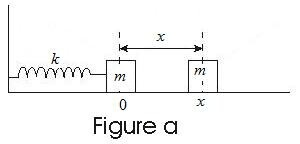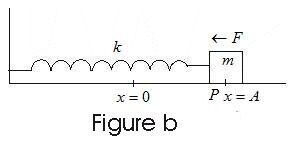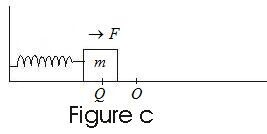# Simple Harmonic Motion (SHM)

### 1. Periodic motion

• If a particle moves such that it repeats its path regularly after equal intervals of time , it's motion is said to be periodic.
• The interval of time required to complete one cycle of motion is called time period of motion.
• If a body in periodic motion moves back and forth over the same path then the motion is said to be viberatory or oscillatory.
• Examples of such motion are to and fro motion of pendulum , viberations of a tuning fork , mass attached to a spring and many more.
• Every oscillatory motion is periodic but every periodic motion is not oscillatory for example motion of earth around the sun is periodic but not oscillatory.
• Simple Harmonic Motion (or SHM) is the simplest form of oscillatory motion.
• SHM arises when force on oscillating body is directly proportional to the displacement from it's equilibrium position and at any point of motion , this force is directed towards the equilibrium position.

### 2. Simple Harmonic Motion (or SHM)

• SHM is a particular type of motion very common in nature.
• In SHM force acting on the particle is always directed towards a fixed point known as equilibrium position and the magnitude of force is directly proportional to the displacement of particle from the equilibrium position and is given by
•      F= -kx
where k is the force constant and negative sign shows that fforce opposes increase in x.
• This force os known as restoring force which takes the particle back towards the equilibrium position , and opposes increase in displacement.
• S.I. unit of force constant k is N/m and magnitude of k depends on elastic properties of system under consideration.
• For understanding the nature of SHM consider a block of mass m whose one end is attached to a spring and another end is held stationary and this block is placed on a smooth horizontal surface shown below in the fig.• Motion of the body can be described with coordinate x taking x=0 i.e. origin as the equilibrium positionwhere the spring is neither stretched or compressed.
• We now take the block from it's equilibrium position to a point P by stretching the spring by a distance OP=A and will then release it.
• After we release the block at point P, the restoring force acts on the block towards equilibrium position O and the block is then accelerated from point P towards point O as shown below in the fig.• Now at equilibrium position this restoring force would become zero but the velocity of block increases as it reaches from point P to O.
• When the block reaches point O it's velocity would be maximum and it then starts to move towards left of equilibrium position O.
• Now this time while going to the left of equilibrium position spring is compressed and the block moves to the point Q where it's velocity becomes zero.• The compressed spring now pushes the block towards the right of equilibrium position where it's velocity increases upto point O and decreases to zero when it reaches point P.
• This way the block oscillates to and fro on the frictionless surface between points P and Q.
• If the distance travelled on both sides of equilibrium position are equal i.e. , OP=OQ then the maximum displacement on either sides of equilibrium are called the Amplitude of oscillations.